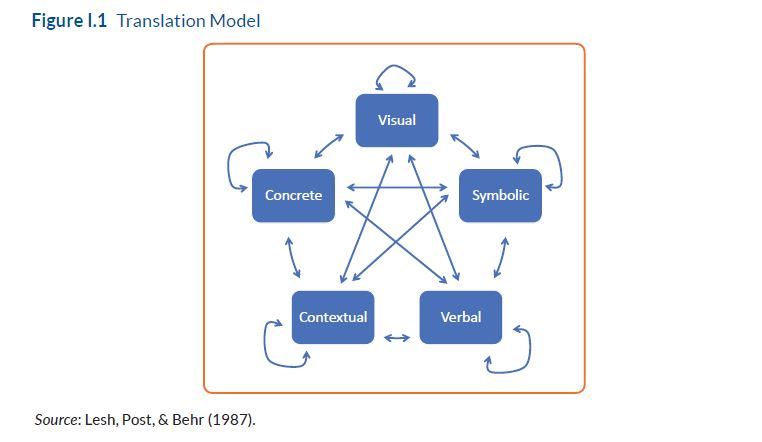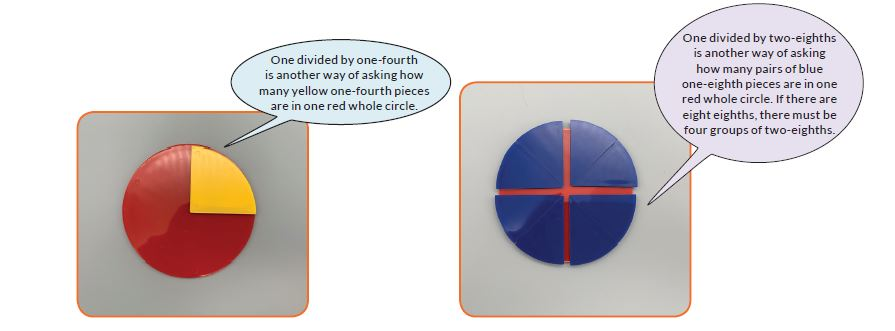Saturday / December 9

# 3 Strategies for Learning Mathematics with ManipulativesAfter two years of interrupted education, we are all working to support students building deep conceptual understanding of mathematics. There’s consensus that using manipulatives to teach mathematics is an important component of success. There’s also consensus that it’s a challenge. Teachers often make comments like these when we talk about teaching with manipulatives:

• How do we move from playing with the manipulatives to learning with them?
• There’s so much stuff to manage!
• It feels like manipulatives are an activity then we get back to the real math.

The key thing to remember is that manipulatives are one of five representations for mathematics. Learning doesn’t come solely from doing an activity with manipulatives. It comes from translating across the representations.Not only can we represent mathematical ideas and actions with manipulatives or other physical objects, we can also use visual representations (e.g., sketches, charts, or graphs), symbolic representations (e.g., equations), verbal language (e.g., knowing that n x n x n, representing volume of a cube, is read as “n by n by n”), and context (when would I use this?). It’s essential that we use all of these in teaching mathematics.  The learning power is in connecting different representations of a problem to each other.

If we incorporate specific strategies for connecting representations into our instructional routines, we move from playing with manipulatives to learning with them. We make the work with manipulatives an integral part of learning rather than a fun activity to break up the “real math.” We start to see students learning through manipulative-based experiences, which makes it worth it to manage the materials and use them more often. Here are three strategies to get you started: Make a Sketch, Caption Your Picture, and Create a Diagram.

### Make a Sketch Strategy

In this strategy, students connect their physical representation with a visual one by making a sketch of their manipulatives. For example, students might spill a cup of two-color counters to represent decomposing a number in multiple ways. Students can sketch the result of each spill.As students create sketches, ask deliberate questions designed to help them connect the physical representation with the visual one. Deep mathematical learning is in these connections – recognizing that the number 7 can be decomposed in many different ways, for example. Questions like these support students making connections between work with the counters and their sketch.

• How many counters are in your cup?
• How many red counters are there? How many yellow?
• How many counters did you draw? Why is that the same as the number of counters in your cup?

Once students are comfortable making sketches, ask them to caption their sketch. A caption is more than a title; it’s a brief description of what’s happening in the image. Captions are traditionally written, but young learners might use technology to record an audio caption or create a video.Captions create connections between physical and verbal representations. They support students in describing their work. This develops mathematical vocabulary and helps students begin to generalize. Deep learning comes not from creating the physical model but from describing what is happening in the model. In the example here, students are using “how many of these are in that” reasoning to understand division involving fractions.

### Create a Diagram Strategy

It’s helpful to think of diagrams as more formal sketches. Diagrams are still visual; students are connecting physical representations made with manipulatives to visual images. Diagrams are often more formal mathematical constructs – a number line or a graph, for example – not simply a sketch of the physical objects. Creating diagrams does not simply preserve the manipulative-based thinking; it creates a representation with mathematical value of its own.

Open arrays are mathematical diagrams, which have value in representing and reasoning about multiplication. In the example below, students are connecting a physical representation of two-digit multiplication with an open array diagram. This same diagram will be useful for multiplying binomials in algebra class.As students create their diagrams, help them see the connection between the physical model and the diagram by asking questions. In this example, ask about the factors and how they are decomposed:

• How is 22 built in this diagram? What about 11? How does this help you multiply?
• Where do you see each of the partial products (200, 20, 20, and 2) in a representation of this multiplication using a different algorithm?
• How might you use this diagram to multiply if you don’t have enough base ten blocks to build the problem?

Use one or more of these strategies to support your students to connect their work with manipulatives to other mathematical representations. In many cases, our goal is success with abstract or symbolic representations. Those have meaning and make sense in relationship to other representations – it looks like this (object or picture) and I can use it in this context. We give every student access to mathematics, and opportunities for agency, when we support them to see mathematics through multiple representations.

#### Tags

Sara Delano Moore is an independent educational consultant at SDM Learning. A fourth-generation educator, her work focuses on helping teachers and students understand mathematics as a coherent and connected discipline through the power of deep understanding and multiple representations for learning. Her interests include building conceptual understanding of mathematics to support procedural fluency and applications, incorporating engaging and high-quality literature into mathematics & science instruction, and connecting mathematics with engineering design in meaningful ways. Sara has worked as a classroom teacher of mathematics and science in the elementary and middle grades, a mathematics teacher educator, Director of the Center for Middle School Academic Achievement for the Commonwealth of Kentucky, and Director of Mathematics & Science at ETA hand2mind. She is a frequent presenter at state, national, and international conferences in mathematics, science, and literacy including the National Council of Teachers of Mathematics (NCTM) & National Council of Supervisors of Mathematics (NCSM) conferences. Her journal articles appear in Mathematics Teaching in the Middle School, Teaching Children Mathematics, Science & Children, and Science Scope. Sara earned her BA in Natural Sciences from The Johns Hopkins University, her M. St. in General Linguistics and Comparative Philology from the University of Oxford (UK), and her Ph.D. in Educational Psychology from the University of Virginia. Learn more about Sara’s education and experience at sdmlearning.com. Follow her on Twitter @saradelanomoore.